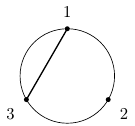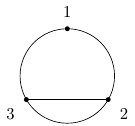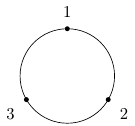Queries for Set partitions: search statistic / browse statistics / browse maps from / browse maps to

# 1. Definition & Example

• A set partition of size $n$ is a partition of the set $\mathcal{S} = \{1,\ldots,n\}$. This is a collection of non-empty pairwise disjoint subsets (parts) of $\mathcal{S}$ whose union is $\mathcal{S}$. In symbols, $\mathcal{P} = \{P_1,\ldots,P_k \}$ such that

$$S = P_1 \sqcup P_2 \sqcup \dots \sqcup P_k, \quad P_i \cap P_j = \emptyset \text{ for all }i \neq j, \quad \emptyset \notin \mathcal{P}.$$

 the 5 Set partitions of size 3{{1,2,3}} {{1,2},{3}} {{1,3},{2}} {{1},{2,3}} {{1},{2},{3}}
• Set partitions of size $n$ are graphically represented by drawing the numbers $1$ through $n$ around a circle and then drawing the convex hulls of the blocks.

• The number of set partitions of size $n$ is $n$-th Bell number $B_n$ (A000110).

• The number of set partitions of size $n$ into $k$ blocks is the Stirling number of the second kind (A008277).

# 2. Additional information

• The set partitions of size $n$ form a poset by containment order. This poset is indeed a lattice which is the intersection lattice of the braid arrangement.

• A set partition is said to be non-crossing if the graphical representation does not have any crossing blocks. In symbols, this is to say that there does not exist $P_i, P_j \in \mathcal{P}$ which contains elements $a, b \in P_i$ and $x, y \in P_j$ such that $a < x < b < y$. The number of non-crossing set partitions of size $n$ is the n-th Catalan number.

# 5. Technical information for database usage

• A set partition is represented as a set of disjoint blocks, which are themselves sets.
• Set partitions are graded by the size of the ground set.
• The database contains all set partitions of size at most 7.